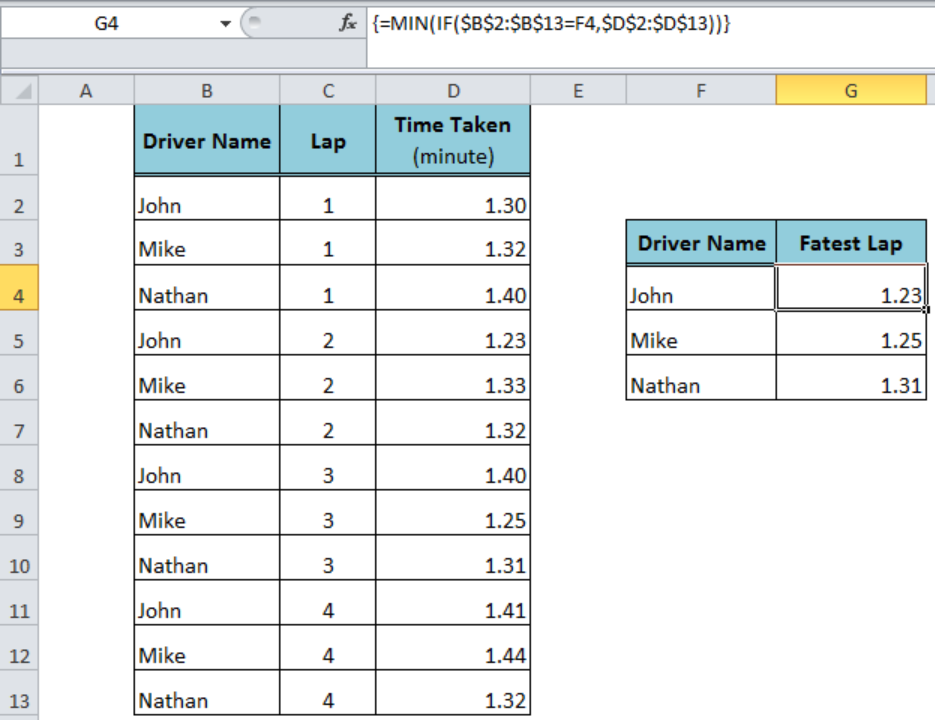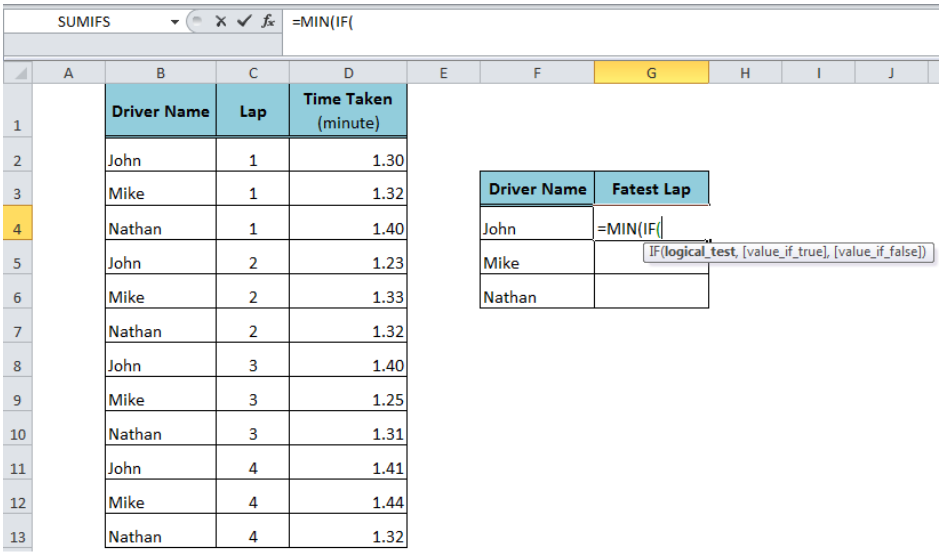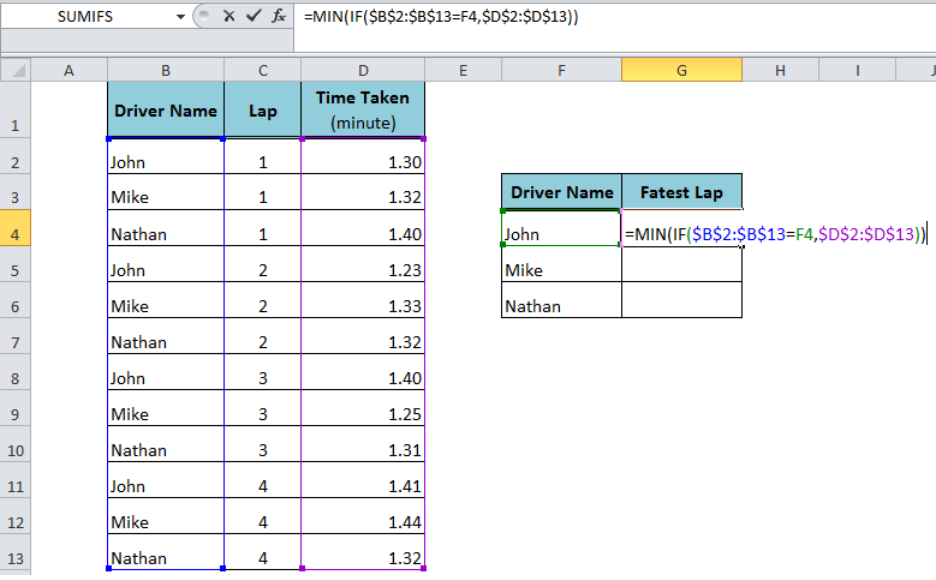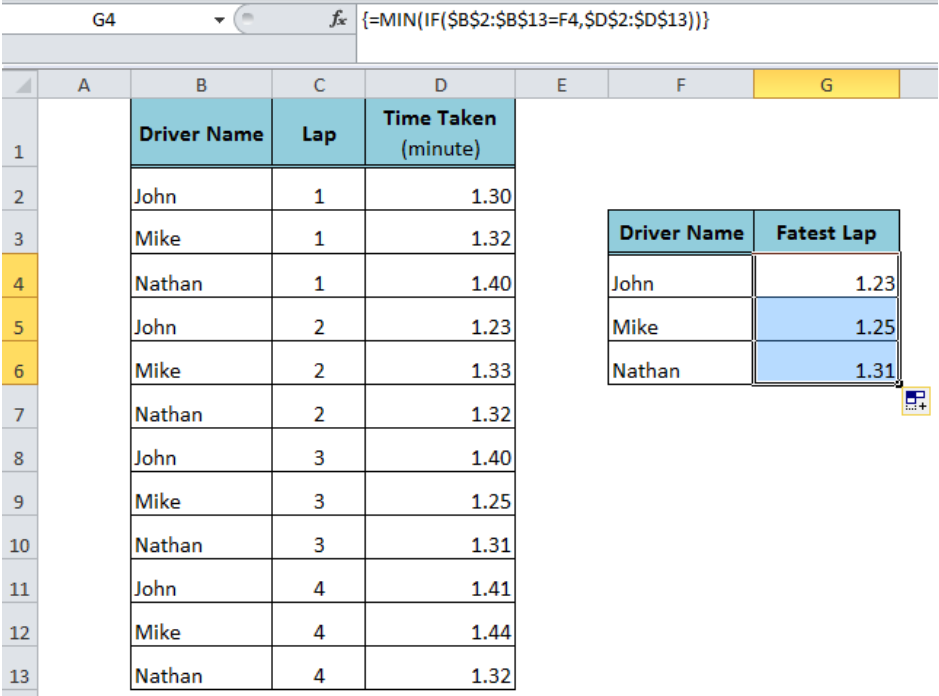Get instant live expert help with Excel or Google Sheets“My Excelchat expert helped me in less than 20 minutes, saving me what would have been 5 hours of work!”

#### Post your problem and you'll get expert help in seconds

Your message must be at least 40 characters
Our professional experts are available now. Your privacy is guaranteed.

# How to Get Minimum Value If Criteria Match in Excel

To return the lowest value from a range of cells we use the Excel MIN function. But, if we need to get the minimum value based on criteria then Excel does not have a built-in function for that. However, we can return the minimum value if criteria match by using the MIN and IF functions together in an array formula.Figure 1. Get Minimum Value If Criteria Match

## Using the MIN and IF Functions in an Array Formula

In order to get the minimum value based on criteria, we need to test the criteria on the criteria range in the logical_test argument and insert the range of values, from which we want to get the minimum value, in the value_if_true argument of the IF function inside the MIN function as per the following syntax;

`=MIN(IF(logical_test, value_if_true))`Figure 2. The Syntax of MIN and IF Formula

In our example, we need to get the minimum value of time taken in a lap (Fastest Lap) by a specified driver as a criteria value. The criteria range containing the names of drivers is B2: B13, the criteria value is given in cell F4 and the range of values is D2: D13. Using the following formula, we can get the minimum value if criteria match and press Ctrl+Shift+Enter keys to make it an array formula;

`=MIN(IF(\$B\$2:\$B\$13=F4,\$D\$2:\$D\$13))`Figure 3. Applying the MIN and IF Formula

Copy or drag the fill handle down to get the results for other criteria values.Figure 4. Final Output of the MIN and IF Formula

## Instant Connection to an Expert through our Excelchat Service

Most of the time, the problem you will need to solve will be more complex than a simple application of a formula or function. If you want to save hours of research and frustration, try our live Excelchat service! Our Excel Experts are available 24/7 to answer any Excel question you may have. We guarantee a connection within 30 seconds and a customized solution within 20 minutes.

### Did this post not answer your question? Get a solution from connecting with the expert.Another blog reader asked this question today on Excelchat:## Subscribe to Excelchat.coAnother blog reader asked this question today on Excelchat: